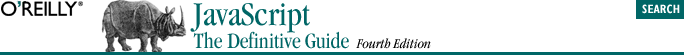home | O'Reilly's CD bookshelfs | FreeBSD | Linux | Cisco | Cisco Exam## 6.3. if

```if (expression)
statement```

In this form, expression is evaluated. If the resulting value is true or can be converted to true, statement is executed. If expression is false or converts to false, statement is not executed. For example:

```if (username == null)       // If username is null or undefined,
username = "John Doe";  // define it```

Or similarly:

```// If username is null, undefined, 0, "", or NaN, it converts to false,
// and this statement will assign a new value to it.

As mentioned in the previous section, we can always replace a single statement with a statement block. So the if statement might also look like this:

```if ((address == null) || (address == "")) {
}```
```if (expression)
statement1
else
statement2```

In this form of the statement, expression is evaluated, and if it is true, statement1 is executed; otherwise, statement2 is executed. For example:

```if (username != null)
else {
}```
```i = j = 1;
k = 2;
if (i == j)
if (j == k)
document.write("i equals k");
else
document.write("i doesn't equal j");    // WRONG!!```

In this example, the inner if statement forms the single statement allowed by the syntax of the outer if statement. Unfortunately, it is not clear (except from the hint given by the indentation) which if the else goes with. And in this example, the indenting hint is wrong, because a JavaScript interpreter actually interprets the previous example as:

```if (i == j) {
if (j == k)
document.write("i equals k");
else
document.write("i doesn't equal j");    // OOPS!
}```

The rule in JavaScript (as in most programming languages) is that an else clause is part of the nearest if statement. To make this example less ambiguous and easier to read, understand, maintain, and debug, you should use curly braces:

```if (i == j) {
if (j == k) {
document.write("i equals k");
}
}
else {  // What a difference the location of a curly brace makes!
document.write("i doesn't equal j");
}```

Although it is not the style used in this book, many programmers make a habit of enclosing the bodies of if and else statements (as well as other compound statements, such as while loops) within curly braces, even when the body consists of only a single statement. Doing so consistently can prevent the sort of problem just shown.